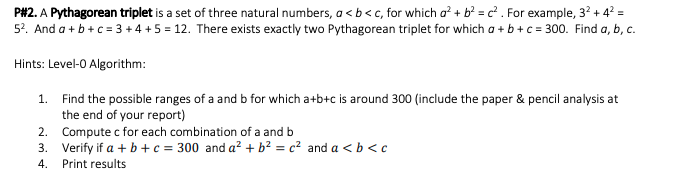# (Solved) : P 2 Pythagorean Triplet Set Three Natural Numbers Q41423910 . . .

Please submit in Python Language, thanks!!P#2. A Pythagorean triplet is a set of three natural numbers, a<b<c, for which o? + b2 = c. For example, 32 +42 = 52. And a + b + c = 3 + 4 + 5 = 12. There exists exactly two Pythagorean triplet for which a + b + c = 300. Find a, b, c. Hints: Level-0 Algorithm: 1. Find the possible ranges of a and b for which a+b+c is around 300 (include the paper & pencil analysis at the end of your report) 2. Compute c for each combination of a and b 3. Verify if a + b + c = 300 and a² + b2 = c2 and a <b<c 4. Print results Show transcribed image text P#2. A Pythagorean triplet is a set of three natural numbers, a